# DAV Class 8 Maths Chapter 1 Worksheet 3 Solutions

The DAV Class 8 Maths Book Solutions and DAV Class 8 Maths Chapter 1 Worksheet 3 Solutions of Squares and Square Roots offer comprehensive answers to textbook questions.

## DAV Class 8 Maths Ch 1 WS 3 Solutions

Question 1.
Find the square root of the following by prime factorisation:
(i) 225
(ii) 441
(iii) 529
(iv) 40000
(v) 7744
(vi) 8281
(vii) 4096
(viii) 28900
Solution:
(i) Resolving 225 into prime factors.225 = 3 × 3 × 5 × 5
∴ $$\sqrt{225}$$ = 3 × 5 = 15

(ii) Resolving 441 into prime factors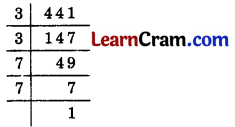441 = 3 × 3 × 7 × 7
∴ $$\sqrt{441}$$ = 3 × 7 = 21

(iii) Let us resolve 529 into prime factors.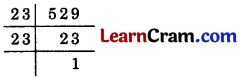529 = 23 × 23
∴ $$\sqrt{529}$$ = 23(iv) Let us resolve 40000 into prime factors40000 = 2 × 2 × 2 × 2 × 2 × 2 × 5 × 5 × 5 × 5
∴ $$\sqrt{40000}$$ = 2 × 2 × 2 × 5 × 5 = 200

(v) Resolving 7744 into prime factors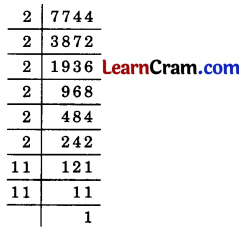7744 = 2 × 2 × 2 × 2 × 2 × 2 × 11 × 11
∴ $$\sqrt{7744}$$ = 2 × 2 × 2 × 11 = 88

(vi) Resolving 8281 into prime factors8281 = 7 × 7 × 13 × 13
∴ $$\sqrt{8281}$$ = 7 × 13 = 91

(vii) Resolving 4096 into prime factors4096 = 2 × 2 × 2 × 2 × 2 × 2 × 2 × 2 × 2 × 2 × 2 × 2
∴ $$\sqrt{4096}$$ = 2 × 2 × 2 × 2 × 2× 2 = 64

(viii) Resolving 28900 into prime factors28900 = 2 × 2 × 5 × 5 × 17 × 17
∴ $$\sqrt{28900}$$ = 2 × 5 × 17 = 170.Question 2.
Find the smallest number by which 1100 must be multiplied so that the product becomes a perfect square. Also, find the square root of the perfect square so obtained.
Solution:
Resolving 1100 into prime factors1100 = 2 × 2 × 5 × 5 × 11
Here, 11 is unpaired. So 11 must be multiplied to 1100 to obtain a perfect square number.
∴ The perfect square number so obtained = 1100 × 11 = 12100

Question 3.
By what smallest number must 180 be multiplied so that it becomes a perfect square?
Also find the square root of the number so obtained.
Solution:
Resolving 180 into prime factors.180 = 2 × 2 × 3 × 3 × 5
Here 5 is unpaired.
∴ 5 is required to multiply 180 to make it perfect square.
∴ Required number is 5 × 180 = 900
∴ $$\sqrt{900}$$ = 2 × 2 × 3 × 3 × 5 × 5
= 2 × 3 × 5 = 30

Question 4.
Find the smallest number by which 3645 must be divided so that it becomes a perfect square. Also, find the square root of the resulting number.
Solution:
Resolving 3645 into prime factors.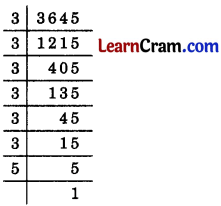3645 = 3 × 3 × 3 × 3 × 3 × 3 × 5
Here 5 is unpaired. So the given number 3645 must be divided by 5 to obtain a perfect square.
So the required number = 3645 ÷ 5 = 729
∴ $$\sqrt{729}$$ = 3 × 3 × 3 × 3 × 3 × 3
= 3 × 3 × 3 = 27Question 5.
A gardener planted 1521 trees in rows such that the number of rows was equal to the number of plants in each row. Find the number of rows.
Solution:
Let the number of rows be x.
number of plants in each row = x
Total number of plants = x × x = x2
∴ x2 = 1521
⇒ x = $$\sqrt{1521}$$⇒ 1521 = 3 × 3 × 13 × 13
⇒ $$\sqrt{1521}$$ = 3 × 13 = 39
Hence the number of rows is 39.

Question 6.
An officer wants to arrange 202500 cadets in the form of a square. How many cadets were there in each row?
Solution:
Let there be x number of cadets in each row.
∴ Number of rows = x
Total number of cadets = x × x = x2
x2 = 202500
x = $$\sqrt{202500}$$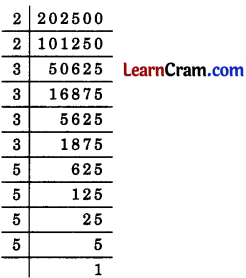202500 = 2 × 2 × 3 × 3 × 3 × 3 × 5 × 5 × 5 × 5
∴ $$\sqrt{202500}$$ = 2 × 3 × 3 × 5 × 5 = 450
Hence, the number of cadets in each row = 450.Question 7.
The area of a square field is 5184 m2. A rectangular field whose length is twice its breadth, has its perimeter equal to the perimeter of the square field. Find the area of the rectangular field.
Solution:
The area of the square field = 5184 m2
∴ side of the square = $$\sqrt{5184}$$ m
Resolving 5184 into prime factors
5184 = 2 × 2 × 2 × 2 × 2 × 2 × 3 × 3 × 3 × 3
∴ $$\sqrt{5184}$$ = 2 × 2 × 2 × 3 × 3 = 72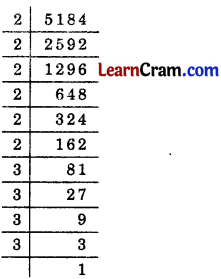∴ Perimeter of the square field = 4 × side
= 4 × 72 = 288 m
Now, let the breadth of the rectangular field be x m.
∴ Length = 2x m
Perimeter of the rectangular field = 2 [l + b] = 2 [2x + x] = 6x m
∴ 6x = 288
⇒ x = $$\frac {288}{6}$$
⇒ x = 48 m.
and length = 2 × 48 = 96 m
∴ Area of the rectangular field = l × b = 96 × 48 = 4608 m2
Hence the required area = 4608 m2

Question 8.
Find the value of $$\sqrt{47089}+\sqrt{24336}$$
Solution:
Resolving 47089 into prime factors,
47089 = 7 × 7 × 31 × 31
∴ $$\sqrt{47089}$$ = 7 × 31 = 217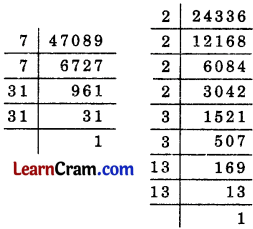Resolving 24336 into prime factors,
24336 = 2 × 2 × 2 × 2 × 3 × 3 × 13 × 13
∴ $$\sqrt{24336}$$ = 2 × 2 × 3 × 13 = 156
Thus, $$\sqrt{47089}+\sqrt{24336}$$ = 217 + 156 = 373.Question 1.
Find the square root of each of the following numbers by prime factorisation.
(i) 130321
(ii) 46656
(iii) 45796
Solution:
(i) Resolving 130321 into prime factors,∴ 130321 = 19 × 19 × 19
7130321 = 19 × 19 = 361

(ii) Resolving 46656 into prime factors,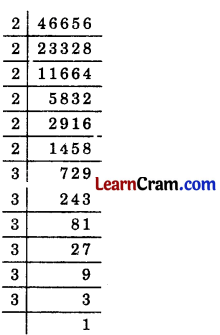46656 = 2 × 2 × 2 × 2 × 2 × 2 × 3 × 3 × 3 × 3 × 3 × 3
746656 = 2 × 2 × 2 × 3 × 3 × 3 = 216

(iii) Resolving 45796 into prime factors,∴ 45796 = 2 × 2 × 107 × 107
$$\sqrt{45796}$$ = 2 × 107 = 214.Question 2.
Is 2352 a perfect square? If not, find the smallest multiple of 2352 which is a perfect square. Find the square root of the number so obtained.
Solution:
Resolving 2352 into prime factors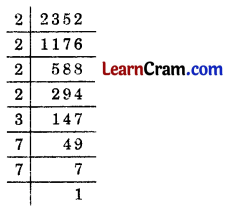∴ 2352 = 2 × 2 × 2 × 2 × 3 × 7 × 7
Here 3 is unpaired. So the given number 2352 is not a perfect square.
∴ 3 is needed to make the given number 2352 as a perfect square.
Hence the smallest multiple of 2352 is 3 which makes it a perfect square.
∴ Perfect square number = 2352 × 3 = 7056
7056 = 2 × 2 × 2 × 2 × 3 × 3 × 7 × 7
$$\sqrt{7056}$$ = 2 × 2 × 3 × 7 = 84

Question 3.
Find the smallest number by which 1008 must be multiplied to make it 2 252 a perfect square. 2 126
Solution:
Resolving 1008 into prime factors,∴ 1008 = 2 × 2 × 2 × 2 × 3 × 3 × 7
Hence, 7 is unpaired. So 7 is required to make a pair of 7 which is the 7 smallest number to make the given number 1008 a perfect square. Hence, 7 is the required number.

Question 4.
Find the smallest number by which 2645 must be divided so that it 23 529 becomes a perfect square. Also, find the square root of the resulting 23 23 number.
Solution:
Resolving 2645 into prime factors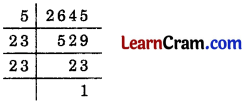∴ 2645 = 5 × 23 × 23
Here 5 has no pair. So the given number 2645 must be divided by 5 to make it a perfect square.
∴ Resulting number = 2645 ÷ 5 = 529
∴ $$\sqrt{529}$$ = 23Question 5.
2025 plants are to be planted in a garden in such a way that each row contains as many plants as the number of rows. Find the number of rows and the number of plants in each row.
Solution:
Let number of rows be x
∴ Number of plants in each row = x
Total number of plants = x × x = x2
∴ x2 = 2025
⇒ x = $$\sqrt{2025}$$
Resolving 2025 into prime factors,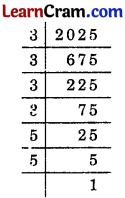2025 = 3 × 3 × 3 × 3 × 5 × 5
$$\sqrt{2025}$$ = 3 × 3 × 5 = 45
Hence the number of plants in each row = 45
and number of rows = 45

Question 6.
Find the smallest square number which is divisible by each of the numbers 6, 9 and 15.
Solution:
The smallest number divisible by 6, 9 and 15 is their L.C.M. which is equal to 2 × 3 × 3 × 5 = 90
Here prime factors are 90 = 2 × 3 × 3 × 5
2 and 5 are unpaired. So 90 is not a perfect square.
∴ 2 × 5 = 10 is needed to multiply 90 to make it a prefect square.
The required smallest number = 90 × 10 = 900.

Question 7.
Find the length of the side of a square whose area = 1024 m2.
Solution:
Area of a square of side x m = x2 m2
∴ x2 = 1024
⇒ x = $$\sqrt{1024}$$
Resolving 1024 into prefect square,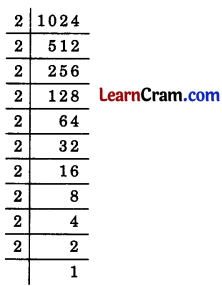1024 = 2 × 2 × 2 × 2 × 2 × 2 × 2 × 2 × 2 × 2
∴ $$\sqrt{1024}$$ = 2 × 2 × 2 × 2 × 2 = 32
Hence, the length of the side of a square = 32 m.Question 8.
The area of a square field is 256 m2 whose perimeter is equal to the perimeter of a rectangular field whose length is thrice its breadth. Find the dimensions of the rectangular field.
Solution:
Area of the square field = 256 m2
∴ Side of the square field = $$\sqrt{256}$$
Resolving 256 into prime factors,256 = 2 × 2 × 2 × 2 × 2 × 2 × 2 × 2
∴ $$\sqrt{256}$$ = 2 × 2 × 2 × 2 = 16
Side of the square field = 16 m
∴ Its perimeter = 4 × 16 = 64 m.
Now, let x m be the breadth of the rectangular field.
∴ Its length = 3x m
Perimeter = 2[l + b] = 2[3x + x] = 8x m
∴ 8x = 64 ⇒ x = 8 m.
Hence the breadth = 8 m and length = 3 × 8 = 24 m.

Question 9.
In a right ∆ABC, ∠B = 90°. If AB = 20 cm, BC = 21 cm, find AC.
Solution:
From Pythagoras Theorem,AC2 = AB2 + BC2
AC2 = (20)2 + (21)2
= 400 + 441 = 841
∴ AC = $$\sqrt{841}$$
Now resolving 841 into prime factors,841 = 29 × 29
∴ $$\sqrt{841}$$ = 29
Hence AC = 29 m.Question 10.
There are 500 children in a school. For a P.T. drill, they have to stand in such a manner that the number of rows is equal to the number of columns. How many children would be left out in this arrangement?
Solution:
Let number of rows be x.
∴ number of columns = x
Total number of students = x × x = x2
∴ x2 = 500
x = $$\sqrt{500}$$
Resolving 500 into prime factors500 = 2 × 2 × 5 × 5 × 5
∴ $$\sqrt{500}$$ = 2 × 5
Here 5 is unpaired. So, 5 students would be left out in this arrangement.